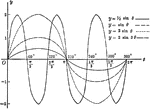### Sine Curves y= sin Ǝ, y= 1/2 sin Ǝ, y=2 sin Ǝ, y= 2 sin 3Ǝ

Sine curves of varying frequency and amplitude plotted from 0 to 2 pi. Graph of y= sin θ, y= 1/2…### Triangle ABC With Height h

Triangle ABC with sides a,b,c labeled and angles A,B,C labeled and h labeled as height.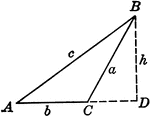### Obtuse Triangle ABC With Height h

Triangle ABC with sides a,b,c labeled and angles A,B,C labeled and h labeled as height.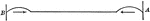### Constructive Interference

"Start a trough from A. At the moment of its reflection as a crest a B, start a crest at A as shown.…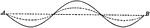### Waves with Different Amplitudes with Equal Periods

"...represents two sets of sound waves with like periods and phases but different amplitudes." -Avery…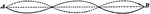### Waves with Equal Periods and Opposite Amplitudes

"...represents two wave systems of equal periods and amplitudes but of opposite phases." -Avery 1895